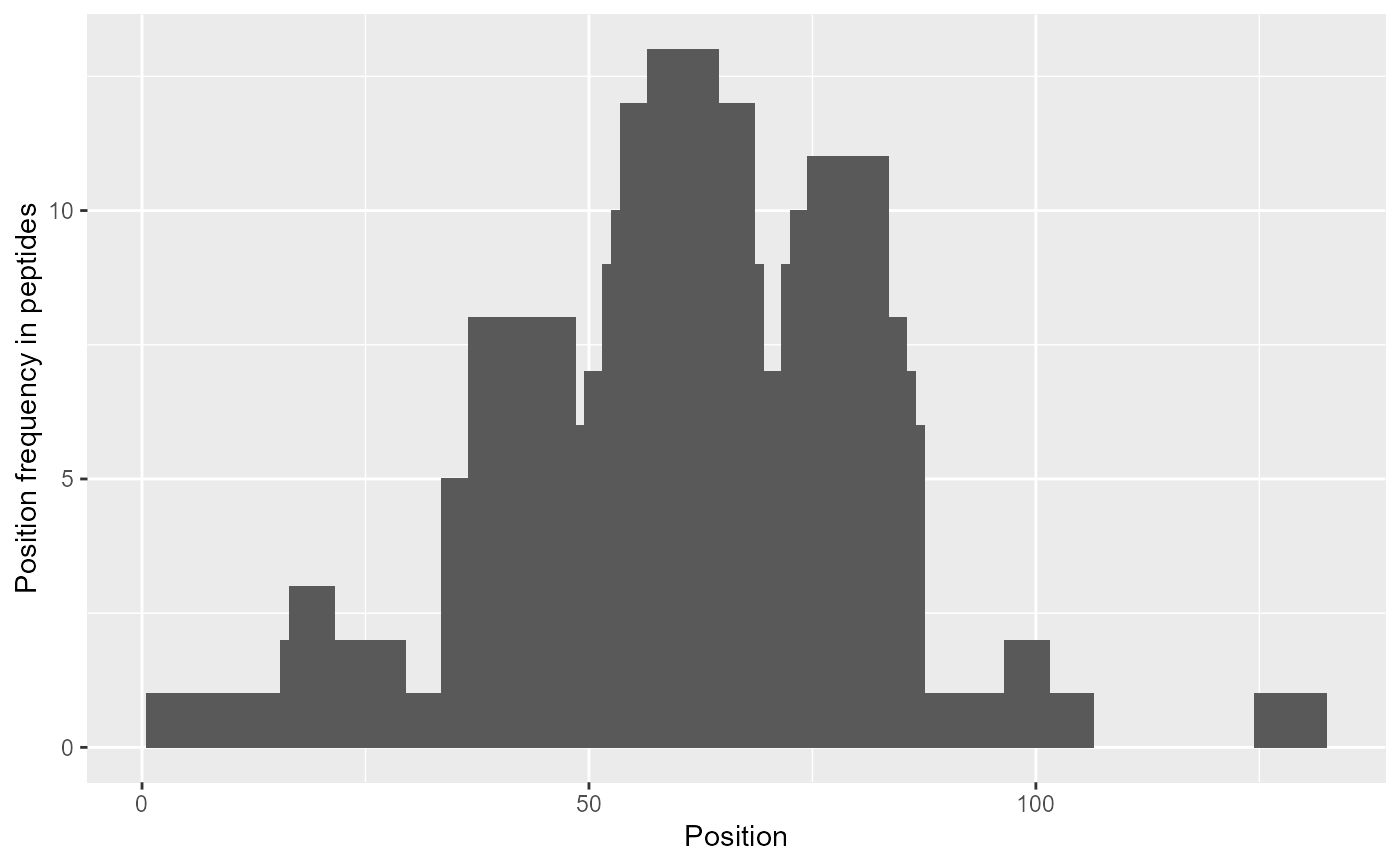Plots the frequency of coverage of protein sequence.

plot_position_frequency(
dat,
protein = dat[["Protein"]],
chosen_state = dat[["State"]]
)

## Arguments

dat data as imported by the read_hdx function protein to be included in plot sequence states to be included in plot

## Value

a ggplot object.

## Details

The function plot_position_frequency plots a histogram of the coverage frequency based on experimental data. The aim of this plot is to see how many times each position of the sequence was covered (by different peptides).

read_hdx plot_coverage

## Examples

# load example data